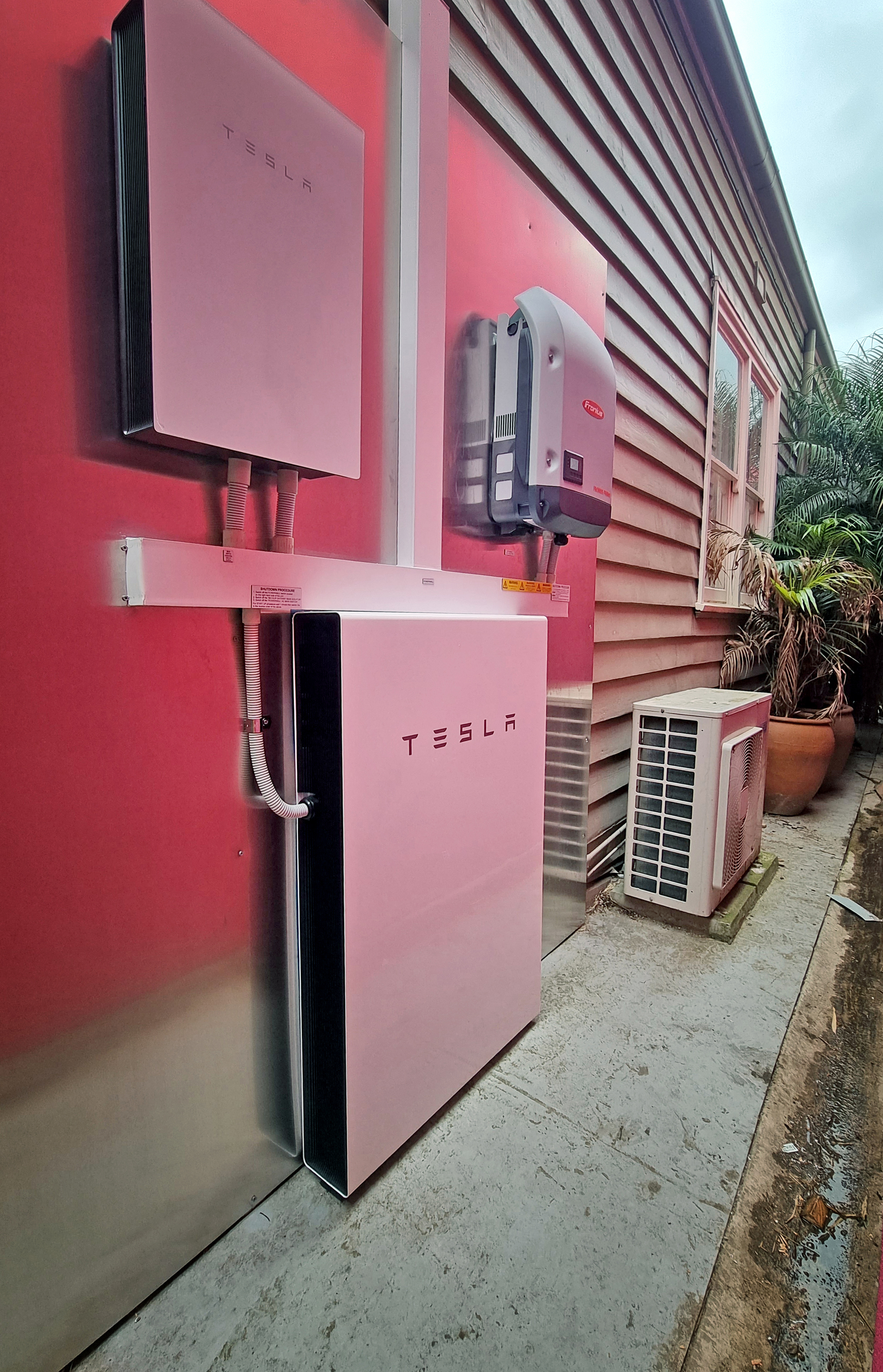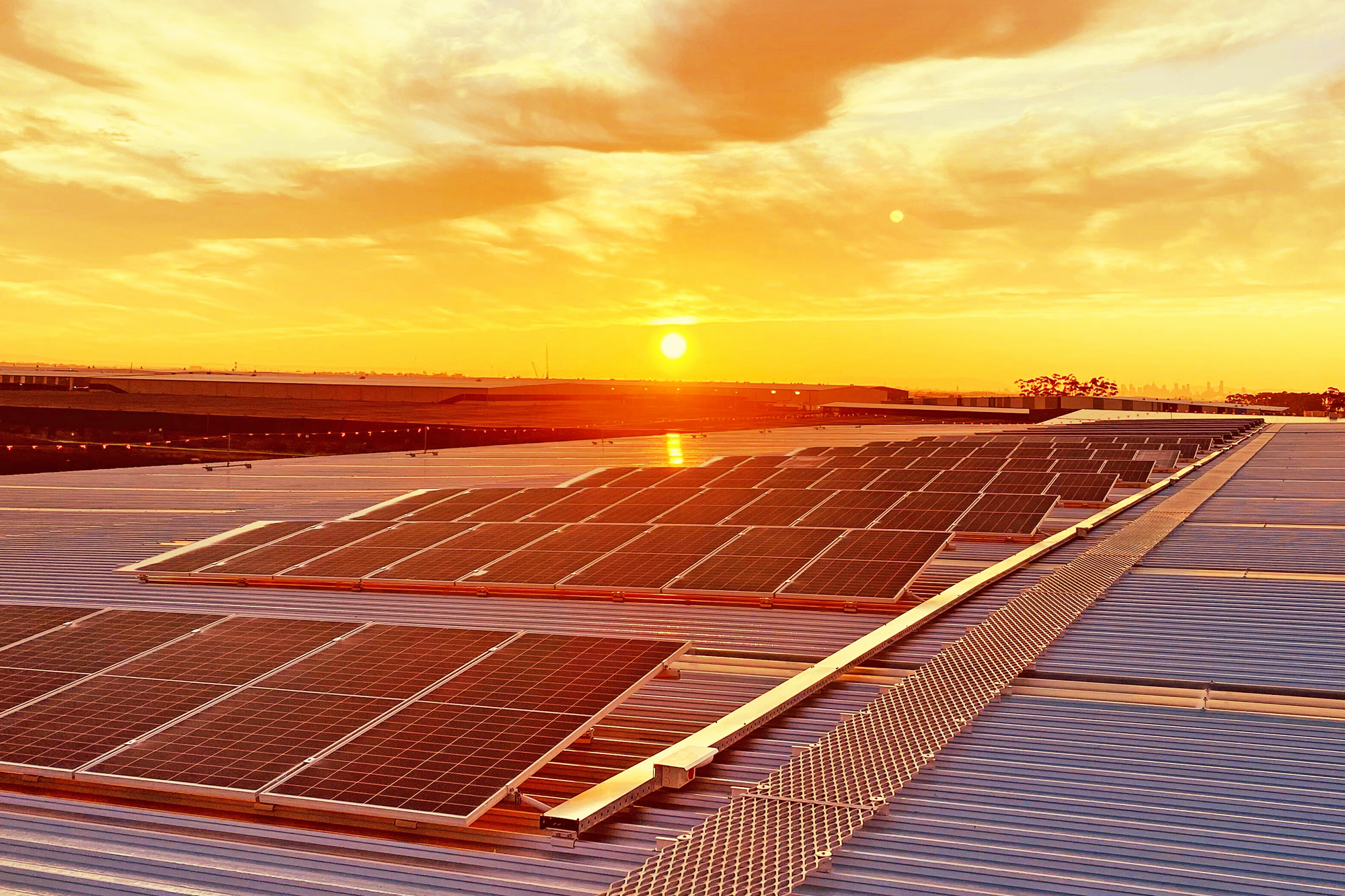# A guide to DC Drop

## What is DC voltage drop?

Volt drop is a decrease of electrical potential when current flows in an electrical circuit and is affected by the properties of the cable that has been selected. In this case, we are talking about the solar DC cable that travels all the way from a collection of photovoltaic panels connected in series all the way back to the inverter.

What cable is selected, what voltage and current  it is being subjected to, how the cable is installed, the ambient temperature and the proximity of existing cables all play a crucial role.

### Why is it important?

The solar panels are the front line in regards to collecting the sun’s energy then converting it into DC where it then travels to the inverter.

Energy that comes out of the solar panels is dissipated due to transmission and is less than the energy available to be converted by the inverter. In other words, there are losses.

This is something that needs to be minimised ideally given that commercial solar systems are a financial proposition.

### What standard refers to DC voltage drop?

As with everything electrical, there are referenced standards and in the case of recommended volt drop on the DC side this is AS/NZ 5033. It recommends a maximum of 3% Volt Drop on the DC side when dealing with solar panel energy transmission.

All standards can be looked at from the perspective of:

Shall:    You must do this, or else

Should: We recommend this particular course of action

The other specific cabling standard is AS/NZ 3008 and anyone involved in the design of any electrical system must be aware of its contents.

### An Example of DC Voltage Drop

Let’s look at a theoretical example to better understand what this means.

Let's say we calculated that there is 60 metres of cable from the panels to the inverter in question. This particular configuration is known as a string and is made up of 19 x solar panels connected in series, i.e the positive of one panel is connected to the negative of the next and so on.

The total voltage at Vmpp ( Voltage at Maximum Power Point) of this series string of 19 panels has been calculated to be 744 and this information is found on the panel’s data sheet.

Vmpp is used as this is the voltage that the solar panel is operating at for the majority of time during the day. If you use Voc ( Voltage at open circuit) or Voc max ( Voc adjusted for temperature) which are both higher figures, your calculations will be out of whack!

### OK, so what now?

So we know that the Vmpp is 744 and AS5033 has kindly suggested, a ‘should’, that the maximum volt drop is 3% so:

744 x 3% = 22.32 Volts, this is the max allowable volt drop for this particular configuration with the cable distance mentioned. We also need the current that the panel produces and in this case I have used the short circuit, Isc of the panel which is 9.51A.

I know, why haven’t you used the current at maximum power point as with the voltage? Well, you can but by using the slightly higher figure you introduce a buffer with your calculations (but this is up to you).

Next on the list is the actual cable selection.

### DC Voltage Drop: Cable Selection

Let's say we decide to use 4mm solar DC cable and this has a sufficient C.C.C. (current carrying capacity) so we now go to the other standard that is specific for cable and that is AS3008. Now this standard is full of tables and can be quite daunting, but persevere.

The calculation I am looking for is the Voltage drop factor, mV / A.m for the cable size in question. This effectively means that for every Amp I send through one metre of the cable there will be a corresponding drop in voltage expressed in millivolts.

Phew. I have selected 4 mm cable at 90 degrees and the result is 10.2 mV /A.m

#### We now have

Cable run: 60 metres

Vmmp: 744

Isc: 9.51

Cable size: 4mm2

Volt drop factor mV / A.m: 10.2

Max allowable Volt drop: 22.32 Volts ( 744 x 3%)

All the ducks are lined up in a row, hopefully.

#### So what is the equation

Basically we take the cable run of 60 metres and multiply it by the Isc which is 9.51A and then multiply by Vc the Volt drop factor which is 10.2:

Vd/r = L x I x Vc/1000 where:

L = length of run, 60 metres

I = current, in this case 9.51 Amps

Vc = Voltage drop factor mV / A.m which is 10.2

#### The result

So voltage drop is;

60 x 9.51 x 10.2/1000 = 5.8 volts

As a % it is 0.78% way less than the 3% recommended by the Standards

You can compare results to an online calculator such as the one below

https://photovoltaic-software.com/solar-tools/dc-ac-drop-voltage-calculator

This is just one of the many ways that can be used to calculate the overall voltage drop. I recommend looking through AS3008 in detail to discover different approaches. The above approach is quite simple and makes certain assumptions.

### Conclusion

Energy transmission involves losses and to minimize these losses it is extremely important to select the right cable that takes into account all the factors mentioned. The cable's method of installation, its CCC (current carrying capacity), the voltage under consideration and other cables in proximity all play a crucial role in the efficient transmission of energy.

At the end of the day what the inverter ultimately receives from the solar panels is tempered by all the factors mentioned above.# Related posts for youTraining
September 22, 2021Training
September 21, 2021

#### Commercial Grid connect signageTraining
September 21, 2021

#### Solar export tariffs! What, more changes?Training
September 13, 2021

#### Over current protection:overload and SC, circuit breakers and fusesTraining
September 13, 2021

#### Heat Pumps and solarTraining
September 13, 2021

#### Satellites, space and solar panels